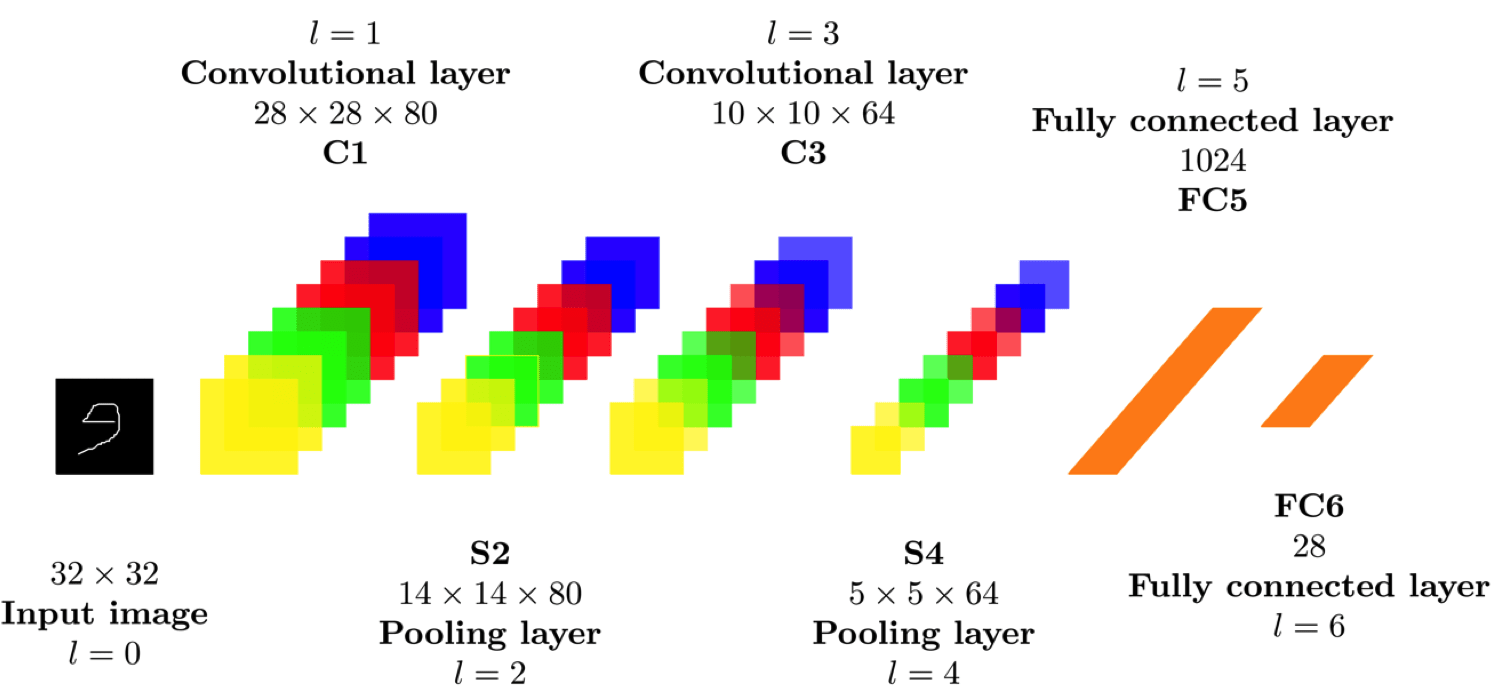## Arabic Handwritten Characters Recognition using Deeplearning4j

### Introduction

Due to the variety of human handwritten styles, the handwritten character recognition system faces some challenges. The authors of the paper (Arabic Handwritten Characters Recognition using Convolutional Neural Network) I built my work on it, introduce a suggested model for the handwritten Arabic character recognition problem using Convolution Neural Network (CNN). They trained their network on a dataset contains 16800 examples of hand written Arabic characters and it gave them an accuracy of 94.9% on testing data.

### Requirements

1. Install Java Development Kit 8 (JDK 8).
2. Install Netbeans.
3. Source code from https://github.com/tahaemara/arabic-characters-recognition.

### Proposed Approach

The proposed CNN design consists of layers as shown in fig. 1 (INPUT → CONV→RELU→POOL→CONV→RELU→ POOL → FC → RELU → FC). "For one direction in a channel (feature map) of the convolutional layer C1, the output is ((32-5) +1) = 28. The max pooling layer S2 has non-overlapping regions, so it down-samples by 2 in each direction, the output is 28/2 = 14. For one direction in a channel (feature map) of the convolutional layer C3, the output is ((14-5) +1 = 10. The max pooling layer S4 has non-overlapping regions, so it down-samples by 2 in each direction, the output is 10/2 = 5 on fourth layer. The output image size of max pooling layer is 5 * 5 = 25. There are 64 channels in the convolutional layer and the same in pooling layer, so the total output of the max pooling layer is 25 * 128 = 1600. This is the size of the input to the fully connected layer FC5. Layer FC6 (Output), contain 28 units and is fully connected to FC5. Finally, the FC6 is composed of softmax classifier to produce 28 output class."See ReferenceFigure 1: The proposed CNN for Arabic handwritten character recognition .

Share: